# Row numbers with nondeterministic order

The SQLPerformance.com bi-weekly newsletter keeps you up to speed on the most recent blog posts and forum discussions in the SQL Server community.

eNews is a bi-monthly newsletter with fun information about SentryOne, tips to help improve your productivity, and much more.

Subscribe

Featured AuthorItzik is a T-SQL trainer, a co-founder of SolidQ, and blogs about T-SQL fundamentals and query tuning.

Itzik’s Posts

The ROW_NUMBER window function has numerous practical applications, well beyond just the obvious ranking needs. Most of the time, when you compute row numbers, you need to compute them based on some order, and you provide the desired ordering specification in the function’s window order clause. However, there are cases where you need to compute row numbers in no particular order; in other words, based on nondeterministic order. This could be across the entire query result, or within partitions. Examples include assigning unique values to result rows, deduplicating data, and returning any row per group.

Note that needing to assign row numbers based on nondeterministic order is different than needing to assign them based on random order. With the former, you just don’t care in what order they are assigned, and whether repeated executions of the query keep assigning the same row numbers to the same rows or not. With the latter, you expect repeated executions to keep changing which rows get assigned with which row numbers. This article explores different techniques for computing row numbers with nondeterministic order. The hope is to find a technique that is both reliable and optimal.

Special thanks to Paul White for the tip concerning constant folding, for the runtime constant technique, and for always being a great source of information!

## When order matters

I’ll use a table called T1 in my examples. Use the following code to create this table and populate it with sample data:

```SET NOCOUNT ON;

USE tempdb;

DROP TABLE IF EXISTS dbo.T1;
GO

CREATE TABLE dbo.T1
(
id INT NOT NULL CONSTRAINT PK_T1 PRIMARY KEY,
grp VARCHAR(10) NOT NULL,
datacol INT NOT NULL
);

INSERT INTO dbo.T1(id, grp, datacol) VALUES
(11, 'A', 50),
( 3, 'B', 20),
( 5, 'A', 40),
( 7, 'B', 10),
( 2, 'A', 50);```

Consider the following query (we’ll call it Query 1):

```SELECT id, grp, datacol,
ROW_NUMBER() OVER(PARTITION BY grp ORDER BY datacol) AS n
FROM dbo.T1;```

Here you want row numbers to be assigned within each group identified by the column grp, ordered by the column datacol. When I ran this query on my system, I got the following output:

```id  grp  datacol  n
--- ---- -------- ---
5   A    40       1
2   A    50       2
11  A    50       3
7   B    10       1
3   B    20       2```

Row numbers are assigned here in a partially deterministic and partially nondeterministic order. What I mean by this is that you do have an assurance that within the same partition, a row with a greater datacol value will get a greater row number value. However, since datacol is not unique within the grp partition, the order of assignment of row numbers among rows with the same grp and datacol values is nondeterministic. Such is the case with the rows with id values 2 and 11. Both have the grp value A and the datacol value 50. When I executed this query on my system for the first time, the row with id 2 got row number 2 and the row with id 11 got row number 3. Never mind the likelihood for this to happen in practice in SQL Server; if I run the query again, theoretically, the row with id 2 could be assigned with row number 3 and the row with id 11 could be assigned with row number 2.

If you need to assign row numbers based on a completely deterministic order, guarantying repeatable results across executions of the query as long as the underlying data doesn’t change, you need the combination of elements in the window partitioning and ordering clauses to be unique. This could be achieved in our case by adding the column id to the window order clause as a tiebreaker. The OVER clause would then be:

`OVER (PARTITION BY grp ORDER BY datacol, id)`

At any rate, when computing row numbers based on some meaningful ordering specification like in Query 1, SQL Server needs to process the rows ordered by the combination of window partitioning and ordering elements. This can be achieved by either pulling the data preordered from an index, or by sorting the data. At the moment there’s no index on T1 to support the ROW_NUMBER computation in Query 1, so SQL Server has to opt for sorting the data. This can be seen in the plan for Query 1 shown in Figure 1.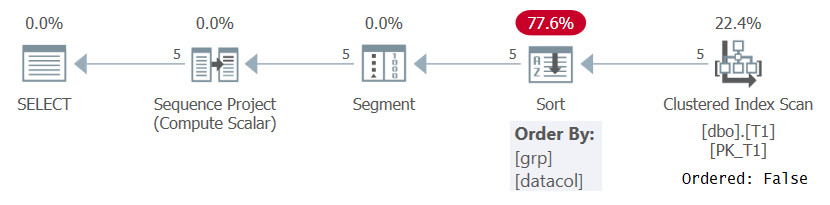Figure 1: Plan for Query 1 without a supporting index

Notice that the plan scans the data from the clustered index with an Ordered: False property. This means that the scan doesn’t need to return the rows ordered by the index key. That’s the case since the clustered index is used here just because it happens to cover the query and not because of its key order. The plan then applies a sort, resulting in extra cost, N Log N scaling, and delayed response time. The Segment operator produces a flag indicating whether the row is the first in the partition or not. Finally, the Sequence Project operator assigns row numbers starting with 1 in each partition.

If you want to avoid the need for sorting, you can prepare a covering index with a key list that is based on the partitioning and ordering elements, and an include list that is based on the covering elements. I like to think of this index as a POC index (for partitioning, ordering and covering). Here’s the definition of the POC that supports our query:

`CREATE INDEX idx_grp_data_i_id ON dbo.T1(grp, datacol) INCLUDE(id);`

Run Query 1 again:

```SELECT id, grp, datacol,
ROW_NUMBER() OVER(PARTITION BY grp ORDER BY datacol) AS n
FROM dbo.T1;```

The plan for this execution is shown in Figure 2.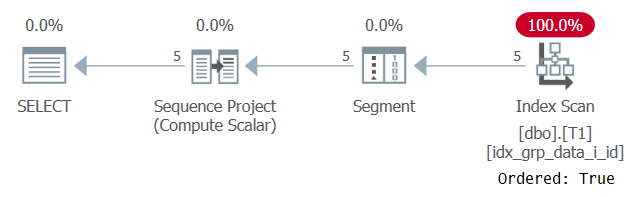Figure 2: Plan for Query 1 with a POC index

Observe that this time the plan scans the POC index with an Ordered: True property. This means that the scan guarantees that the rows will be returned in index key order. Since the data is pulled preordered from the index like the window function needs, there’s no need for explicit sorting. The scaling of this plan is linear and the response time is good.

## When order doesn't matter

Things get a bit tricky when you need to assign row numbers with a completely nondeterministic order. The natural thing to want to do in such a case is to use the ROW_NUMBER function without specifying a window order clause. First, let’s check whether the SQL standard allows this. Here’s the relevant part of the standard defining the syntax rules for window functions:

Syntax Rules

5) Let WNS be the <window name or specification>. Let WDX be a window structure descriptor that describes the window defined by WNS.

6) If <ntile function>, <lead or lag function>, <rank function type> or ROW_NUMBER is specified, then:

a) If <ntile function>, <lead or lag function>, RANK or DENSE_RANK is specified, then the window ordering clause WOC of WDX shall be present.

f) ROW_NUMBER() OVER WNS is equivalent to the <window function>: COUNT (*) OVER (WNS1 ROWS UNBOUNDED PRECEDING)

Notice that item 6 lists the functions <ntile function>, <lead or lag function>, <rank function type> or ROW_NUMBER, and then item 6a says that for the functions <ntile function>, <lead or lag function>, RANK or DENSE_RANK the window order clause shall be present. There’s no explicit language stating whether ROW_NUMBER requires a window order clause or not, but the mention of the function in item 6 and its omission in 6a could imply that the clause is optional for this function. It’s pretty obvious why functions like RANK and DENSE_RANK would require a window order clause, since these functions specialize in handling ties, and ties only exists when there’s ordering specification. However, you could certainly see how the ROW_NUMBER function could benefit from an optional window order clause.

So, let’s give it a try, and attempt to compute row numbers with no window ordering in SQL Server:

```SELECT id, grp, datacol,
ROW_NUMBER() OVER() AS n
FROM dbo.T1;```

This attempt results in the following error:

Msg 4112, Level 15, State 1, Line 53
The function 'ROW_NUMBER' must have an OVER clause with ORDER BY.

Indeed, if you check SQL Server’s documentation of the ROW_NUMBER function, you will find the following text:

“order_by_clause

The ORDER BY clause determines the sequence in which the rows are assigned their unique ROW_NUMBER within a specified partition. It is required.”

So apparently the window order clause is mandatory for the ROW_NUMBER function in SQL Server. That’s also the case in Oracle, by the way.

I have to say that I’m not sure I understand the reasoning behind this requirement. Remember that you are allowing to define row numbers based on a partially nondeterministic order, like in Query 1. So why not allow nondeterminism all the way? Perhaps there’s some reason that I’m not thinking about. If you can think of such a reason, please do share.

At any rate, you could argue that if you don’t care about order, given that the window order clause is mandatory, you can specify any order. The problem with this approach is that if you order by some column from the queried table(s) this could involve an unnecessary performance penalty. When there’s no supporting index in place, you’ll pay for explicit sorting. When there is a supporting index in place, you’re limiting the storage engine to an index order scan strategy (following the index linked list). You don’t allow it more flexibility like it usually has when order doesn’t matter in choosing between an index order scan and an allocation order scan (based on IAM pages).

One idea that is worth trying is to specify a constant, like 1, in the window order clause. If supported, you’d hope that the optimizer is smart enough to realize that all rows have the same value, so there’s no real ordering relevance and therefore no need to force a sort or an index order scan. Here’s a query attempting this approach:

```SELECT id, grp, datacol,
ROW_NUMBER() OVER(ORDER BY 1) AS n
FROM dbo.T1;```

Unfortunately, SQL Server does not support this solution. It generates the following error:

Msg 5308, Level 16, State 1, Line 56
Windowed functions, aggregates and NEXT VALUE FOR functions do not support integer indices as ORDER BY clause expressions.

Apparently, SQL Server assumes that if you’re using an integer constant in the window order clause, it represents an ordinal position of an element in the SELECT list, like when you specify an integer in the presentation ORDER BY clause. If that’s the case, another option that is worthwhile trying is to specify a noninteger constant, like so:

```SELECT id, grp, datacol,
ROW_NUMBER() OVER(ORDER BY 'No Order') AS n
FROM dbo.T1;```

Turns out that this solution is unsupported as well. SQL Server generates the following error:

Msg 5309, Level 16, State 1, Line 65
Windowed functions, aggregates and NEXT VALUE FOR functions do not support constants as ORDER BY clause expressions.

Apparently, the window order clause doesn’t support any kind of constant.

So far we’ve learned the following about the ROW_NUMBER function’s window ordering relevance in SQL Server:

1. ORDER BY is required.
2. Cannot order by an integer constant since SQL Server thinks you're trying to specify an ordinal position in the SELECT.
3. Cannot order by any kind of constant.

The conclusion is that you’re supposed to order by expressions that are not constants. Obviously, you can order by a column list from the queried table(s). But we’re on a quest to find an efficient solution where the optimizer can realize that there’s no ordering relevance.

## Constant folding

The conclusion so far is that you cannot use constants in the ROW_NUMBER’s window order clause, but what about expressions based on constants, such as in the following query:

```SELECT id, grp, datacol,
ROW_NUMBER() OVER(ORDER BY 1+0) AS n
FROM dbo.T1;```

However, this attempt falls victim to a process known as constant folding, which normally has a positive performance impact on queries. The idea behind this technique is to improve query performance by folding some expression based on constants to their result constants at an early stage of the query processing. You can find details on what kinds of expressions can be constant folded here. Our expression 1+0 is folded to 1, resulting in the very same error that you got when specifying the constant 1 directly:

Msg 5308, Level 16, State 1, Line 79
Windowed functions, aggregates and NEXT VALUE FOR functions do not support integer indices as ORDER BY clause expressions.

You would face a similar situation when attempting to concatenate two character string literals, like so:

```SELECT id, grp, datacol,
ROW_NUMBER() OVER(ORDER BY 'No' + ' Order') AS n
FROM dbo.T1;```

You get the same error that you got when specifying the literal 'No Order' directly:

Msg 5309, Level 16, State 1, Line 55
Windowed functions, aggregates and NEXT VALUE FOR functions do not support constants as ORDER BY clause expressions.

## Bizarro world – errors that prevent errors

Life is full of surprises…

One thing that prevents constant folding is when the expression would normally results in an error. For example, the expression 2147483646+1 can be constant folded since it results in a valid INT-typed value. Consequently, an attempt to run the following query fails:

```SELECT id, grp, datacol,
ROW_NUMBER() OVER(ORDER BY 2147483646+1) AS n
FROM dbo.T1;```
Msg 5308, Level 16, State 1, Line 109
Windowed functions, aggregates and NEXT VALUE FOR functions do not support integer indices as ORDER BY clause expressions.

However, the expression 2147483647+1 cannot be constant folded because such an attempt would have resulted in an INT-overflow error. The implication on ordering is quite interesting. Try the following query (we’ll call this one Query 2):

```SELECT id, grp, datacol,
ROW_NUMBER() OVER(ORDER BY 2147483647+1) AS n
FROM dbo.T1;```

Oddly, this query runs successfully! What happens is that on one hand, SQL Server fails to apply constant folding, and therefore the ordering is based on an expression that is not a single constant. On the other hand, the optimizer figures that the ordering value is the same for all rows, so it ignores the ordering expression altogether. This is confirmed when examining the plan for this query as shown in Figure 3.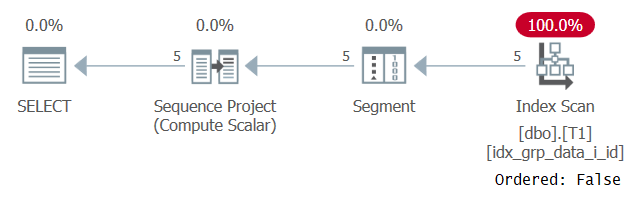Figure 3: Plan for Query 2

Observe that the plan scans some covering index with an Ordered: False property. This was exactly our performance goal.

In a similar manner, the following query involves a successful constant folding attempt, and therefore fails:

```SELECT id, grp, datacol,
ROW_NUMBER() OVER(ORDER BY 1/1) AS n
FROM dbo.T1;```
Msg 5308, Level 16, State 1, Line 123
Windowed functions, aggregates and NEXT VALUE FOR functions do not support integer indices as ORDER BY clause expressions.

The following query involves a failed constant folding attempt, and therefore succeeds, generating the plan shown earlier in Figure 3:

```SELECT id, grp, datacol,
ROW_NUMBER() OVER(ORDER BY 1/0) AS n
FROM dbo.T1;```

The following query involves a successful constant folding attempt (VARCHAR literal '1' gets implicitly converted to the INT 1, and then 1 + 1 is folded to 2), and therefore fails:

```SELECT id, grp, datacol,
ROW_NUMBER() OVER(ORDER BY 1+'1') AS n
FROM dbo.T1;```
Msg 5308, Level 16, State 1, Line 134
Windowed functions, aggregates and NEXT VALUE FOR functions do not support integer indices as ORDER BY clause expressions.

The following query involves a failed constant folding attempt (cannot convert 'A' to INT), and therefore succeeds, generating the plan shown earlier in Figure 3:

```SELECT id, grp, datacol,
ROW_NUMBER() OVER(ORDER BY 1+'A') AS n
FROM dbo.T1;```

To be honest, even though this bizarro technique achieves our original performance goal, I cannot say that I consider it safe and therefore I’m not so comfortable relying on it.

## Runtime constants based on functions

Continuing the search for a good solution for computing row numbers with nondeterministic order, there are a few techniques that seem safer than the last quirky solution: using runtime constants based on functions, using a subquery based on a constant, using an aliased column based on a constant and using a variable.

As I explain in T-SQL bugs, pitfalls, and best practices – determinism, most functions in T-SQL are evaluated only once per reference in the query—not once per row. This is the case even with most nondeterministic functions like GETDATE and RAND. There are very few exceptions to this rule, like the functions NEWID and CRYPT_GEN_RANDOM, which do get evaluated once per row. Most functions, like GETDATE, @@SPID and many others, are evaluated once at the beginning of the query, and their values are then considered runtime constants. A reference to such functions does not get constant folded. These characteristics make a runtime constant that is based on a function a good choice as the window ordering element, and indeed, it seems that T-SQL supports it. At the same time, the optimizer realizes that in practice there’s no ordering relevance, avoiding unnecessary performance penalties.

Here’s an example using the GETDATE function:

```SELECT id, grp, datacol,
ROW_NUMBER() OVER(ORDER BY GETDATE()) AS n
FROM dbo.T1;```

This query gets the same plan shown earlier in Figure 3.

Here’s another example using the @@SPID function (returning the current session ID):

```SELECT id, grp, datacol,
ROW_NUMBER() OVER(ORDER BY @@SPID) AS n
FROM dbo.T1;```

What about the function PI? Try the following query:

```SELECT id, grp, datacol,
ROW_NUMBER() OVER(ORDER BY PI()) AS n
FROM dbo.T1;```

This one fails with the following error:

Msg 5309, Level 16, State 1, Line 153
Windowed functions, aggregates and NEXT VALUE FOR functions do not support constants as ORDER BY clause expressions.

Functions like GETDATE and @@SPID get reevaluated once per execution of the plan, so they can’t get constant folded. PI represents the same constant always, and therefore does get constant folded.

As mentioned earlier, there are very few functions that get evaluated once per row, such as NEWID and CRYPT_GEN_RANDOM. This makes them a bad choice as the window ordering element if you need nondeterministic order—not to confuse with random order. Why pay an unnecessary sort penalty?

Here’s an example using the NEWID function:

```SELECT id, grp, datacol,
ROW_NUMBER() OVER(ORDER BY NEWID()) AS n
FROM dbo.T1;```

The plan for this query is shown in Figure 4, confirming that SQL Server added explicit sorting based on the function’s result.Figure 4: Plan for Query 3

If you do want the row numbers to be assigned in random order, by all means, that’s the technique you want to use. You just need to be aware that it incurs the sort cost.

## Using a subquery

You can also use a subquery based on a constant as the window ordering expression (e.g., ORDER BY (SELECT 'No Order')). Also with this solution, SQL Server’s optimizer recognizes that there’s no ordering relevance, and therefore doesn’t impose an unnecessary sort or limit the storage engine’s choices to ones that must guarantee order. Try running the following query as an example:

```SELECT id, grp, datacol,
ROW_NUMBER() OVER(ORDER BY (SELECT 'No Order')) AS n
FROM dbo.T1;```

You get the same plan shown earlier in Figure 3.

One of the great benefits of this technique is that you can add your own personal touch. Maybe you really like NULLs:

```SELECT id, grp, datacol,
ROW_NUMBER() OVER(ORDER BY (SELECT NULL)) AS n
FROM dbo.T1;```

Maybe you really like a certain number:

```SELECT id, grp, datacol,
ROW_NUMBER() OVER(ORDER BY (SELECT 42)) AS n
FROM dbo.T1;```

Maybe you want to send someone a message:

```SELECT id, grp, datacol,
ROW_NUMBER() OVER(ORDER BY (SELECT 'Lilach, will you marry me?')) AS n
FROM dbo.T1;```

You get the point.

## Doable, but awkward

There are a couple of techniques that work, but are a bit awkward. One is to define a column alias for an expression based on a constant, and then use that column alias as the window ordering element. You can do this either using a table expression or with the CROSS APPLY operator and a table value constructor. Here’s an example for the latter:

```SELECT id, grp, datacol,
ROW_NUMBER() OVER(ORDER BY [I'm a bit ugly]) AS n
FROM dbo.T1 CROSS APPLY ( VALUES('No Order') ) AS A([I'm a bit ugly]);```

You get the same plan shown earlier in Figure 3.

Another option is to using a variable as the window ordering element:

```DECLARE @ImABitUglyToo AS INT = NULL;

SELECT id, grp, datacol,
ROW_NUMBER() OVER(ORDER BY @ImABitUglyToo) AS n
FROM dbo.T1;```

This query also gets the plan shown earlier in Figure 3.

## What if I use my own UDF?

You might think that using your own UDF that returns a constant could be a good choice as the window ordering element when you want nondeterministic order, but it isn’t. Consider the following UDF definition as an example:

```DROP FUNCTION IF EXISTS dbo.YouWillRegretThis;
GO

CREATE FUNCTION dbo.YouWillRegretThis() RETURNS INT
AS
BEGIN
RETURN NULL
END;
GO```

Try using the UDF as the window ordering clause, like so (we’ll call this one Query 4):

```SELECT id, grp, datacol,
ROW_NUMBER() OVER(ORDER BY dbo.YouWillRegretThis()) AS n
FROM dbo.T1;```

Prior to SQL Server 2019 (or parallel compatibility level < 150), user defined functions get evaluated per row. Even if they return a constant, they don’t get inlined. Consequently, on one hand you can use such a UDF as the window ordering element, but on the other hand this results in a sort penalty. This is confirmed by examining the plan for this query, as shown in Figure 5.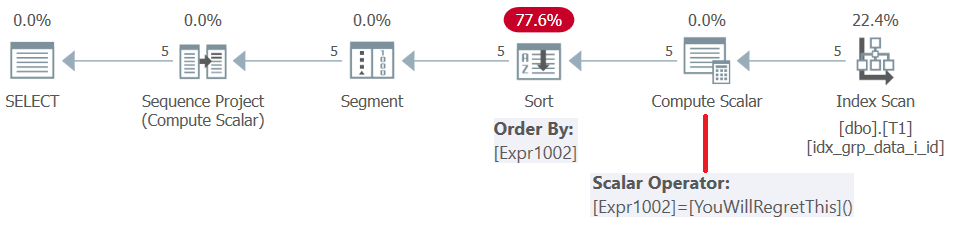Figure 5: Plan for Query 4

Starting with SQL Server 2019, under compatibility level >= 150, such user defined functions get inlined, which is mostly a great thing, but in our case results in an error:

Msg 5309, Level 16, State 1, Line 217
Windowed functions, aggregates and NEXT VALUE FOR functions do not support constants as ORDER BY clause expressions.

So using a UDF based on a constant as the window ordering element either forces a sort or an error depending on the version of SQL Server you’re using and your database compatibility level. In short, don’t do this.

## Partitioned row numbers with nondeterministic order

A common use case for partitioned row numbers based on nondeterministic order is returning any row per group. Given that by definition a partitioning element exists in this scenario, you would think that a safe technique in such a case would be to use the window partitioning element also as the window ordering element. As a first step you compute row numbers like so:

```SELECT id, grp, datacol,
ROW_NUMBER() OVER(PARTITION BY grp ORDER BY grp) AS n
FROM dbo.T1;```

The plan for this query is shown in Figure 6.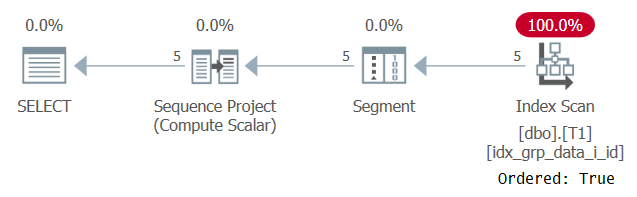Figure 6: Plan for Query 5

The reason that our supporting index is scanned with an Ordered: True property is because SQL Server does need to process each partition’s rows as a single unit. That’s the case prior to filtering. If you filter only one row per partition, you have both order-based and hash-based algorithms as options.

The second step is to place the query with the row number computation in a table expression, and in the outer query filter the row with row number 1 in each partition, like so:

```WITH C AS
(
SELECT id, grp, datacol,
ROW_NUMBER() OVER(PARTITION BY grp ORDER BY grp) AS n
FROM dbo.T1
)
SELECT id, grp, datacol
FROM C
WHERE n = 1;```

Theoretically this technique is supposed to be safe, but Paul white found a bug that shows that using this method you can get attributes from different source rows in the returned result row per partition. Using a runtime constant based on a function or a subquery based on a constant as the ordering element seem to be safe even with this scenario, so make sure you use a solution such as the following instead:

```WITH C AS
(
SELECT id, grp, datacol,
ROW_NUMBER() OVER(PARTITION BY grp ORDER BY (SELECT 'No Order')) AS n
FROM dbo.T1
)
SELECT id, grp, datacol
FROM C
WHERE n = 1;```

## No one shall pass this way without my permission

Trying to compute row numbers based on nondeterministic order is a common need. It would have been nice if T-SQL simply made the window order clause optional for the ROW_NUMBER function, but it doesn’t. If not, it would have been nice if it at least allowed using a constant as the ordering element, but that’s not a supported option either. But if you ask nicely, in the form of a subquery based on a constant or a runtime constant based on a function, SQL Server will allow it. These are the two options that I’m most comfortable with. I don’t really feel comfortable with the quirky erroneous expressions that seem to work so I can’t recommend this option.